Pathogen evolution, selection and immunity
Source code
Contributors
Latest commits
Pages

# Summary statistics of neutral population models

This is all assuming a Wright-Fisher population of discrete non-overlapping generations.

## Parameters

• There are a fixed number of N individuals.

• Mutations enter the population at a rate of μ mutations per individual per site per generation.

• In a haploid population (usually the case for pathogens), we summarize the population with the parameter θ, which equals 2. Without temporally resolved data, it’s not possible to separately estimate N and μ; in most population genetic circumstances, we can only estimate θ. With temporally resolved data we can separate θ into N and μ.

## Diversity

Genetic diversity is most commonly summarized with the statistic π, which is equal to the average number of mutations per site between two random individuals in the population. π is most commonly measured in terms of substitutions per site. The expectation of π follows

$\displaystyle E[\pi] = \theta = 2N\mu$

π for Drosophila and π for flu is approximately 0.01, while π for humans is approximately 0.001. This means that for an average length gene of 1000 basepairs, two random fruit flies or two random flues will probably differ at ~10 sites, while two random humans will differ at ~1 site.

## Unique variants

The number of unique haplotypes in a sample of n sequences can be estimated from Ewen’s sampling formula. Ewen’s sampling formula gives the probability of observing a1 copies of haplotype 1, a2 copies of haplotype 2, etc… in a sample of n sequences. The sole parameter of the sampling formula is θ. Thus θ is sufficient to predict the entire distribution of haplotype frequencies. The expectation of k unique haplotypes follows:

$\displaystyle E[k] = \sum^{n}_{i=1} \frac{\theta}{\theta + i - 1}$

With θ = 0.2, there is usually only a single dominant haplotype in the population.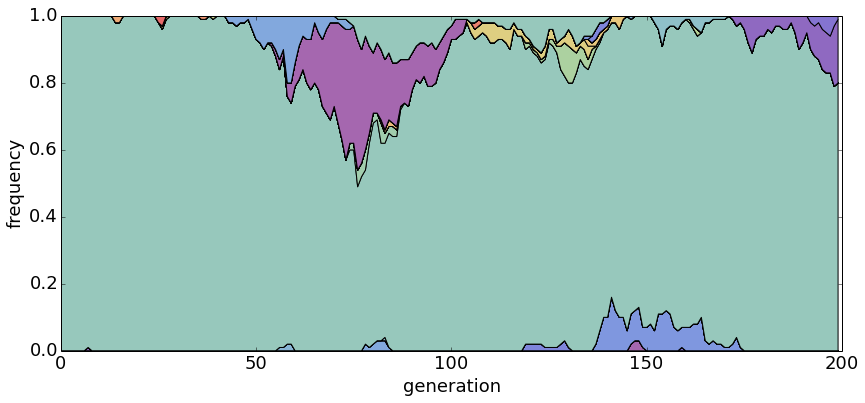With θ = 1.0, there are generally a small handful of haplotypes.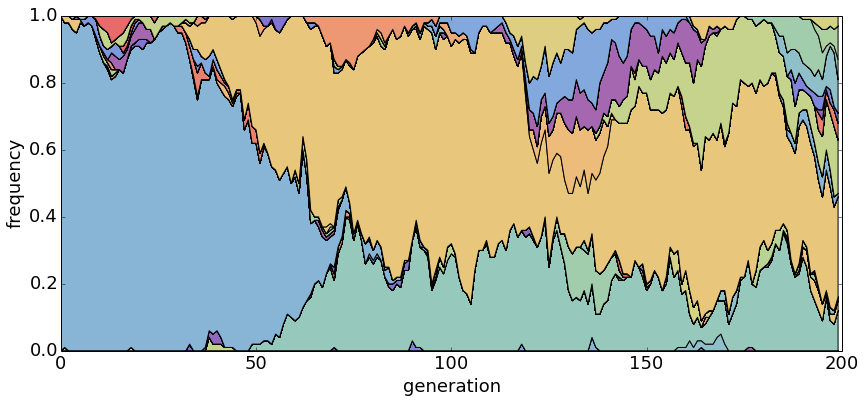With θ = 5.0, there are many segregating haplotypes.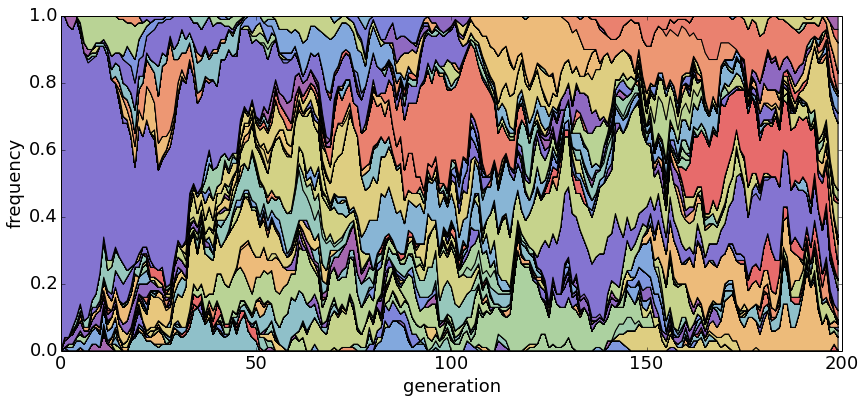## Chance of fixation

A new mutant appears in the population at an initial frequency p of $1/N$. It has a $1/N$ of eventually fixing in the population and a $(N-1)/N$ chance of being lost from the population.

Similarly, if a mutant is at population frequency p, then it has a p chance of fixing. At any point in time, looking forwards, the chance of fixation of a neutral mutation is just its frequency.

## Time to fixation

Conditioned on a neutral mutant fixing, the expected time to fixation is 2N generations. Thus, the rate of population turnover scales inversely with population size. This time to fixation is also a measure of the strength of random genetic drift.

With θ = 1 and N = 20, haplotypes emerge and disappear rapidly.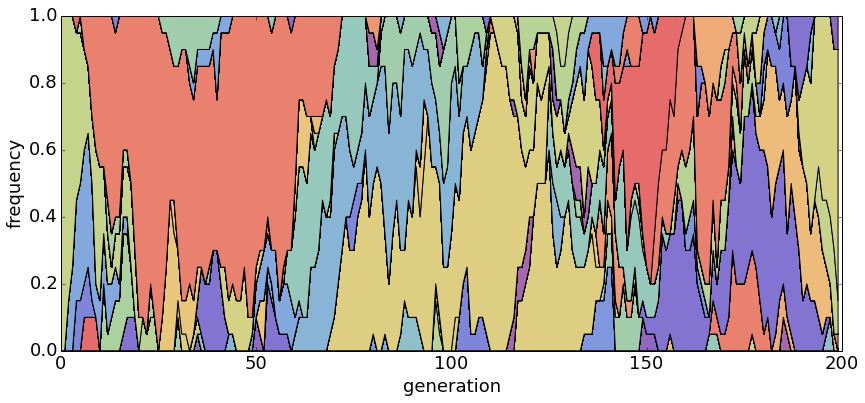With θ = 1 and N = 100, population turnover takes approximately 200 generations.With θ = 1 and N = 500, population turnover occurs slowly.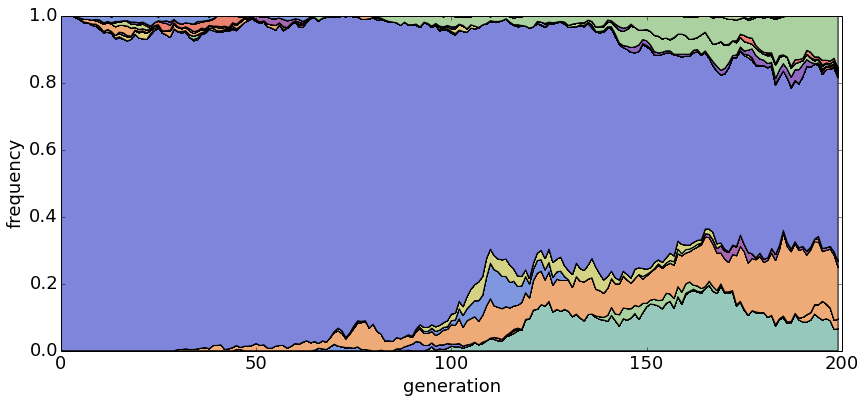## Divergence

Each generation we expect mutations across the entire population. Each mutation has a $1/N$ chance of fixing. Thus the rate of fixed mutations is $N \mu \times 1/N = \mu$.

This result, that the rate of neutral divergence across a species is equal to the rate of mutation in a single individual, is a classic finding from Kimura.

# Overview

Mutation rate μ determines the rate of interspecies divergence, population size N determines the rate of population turnover and their product determines the level of standing genetic variation.